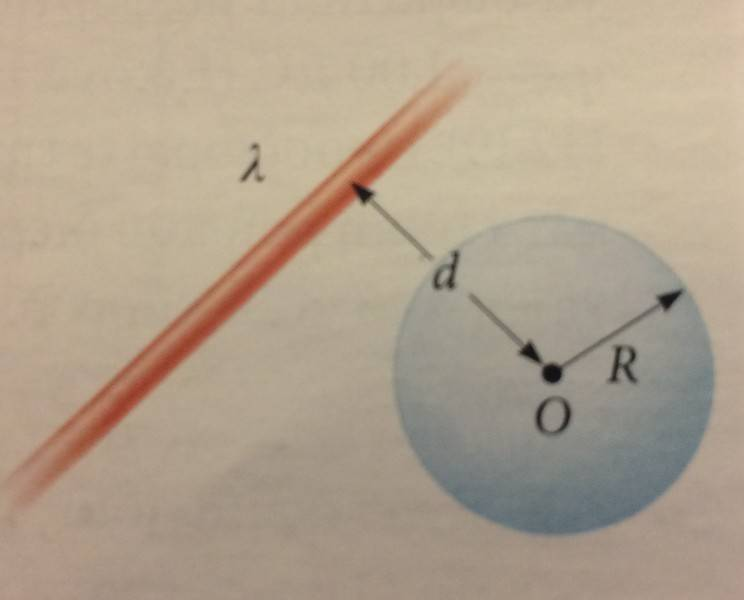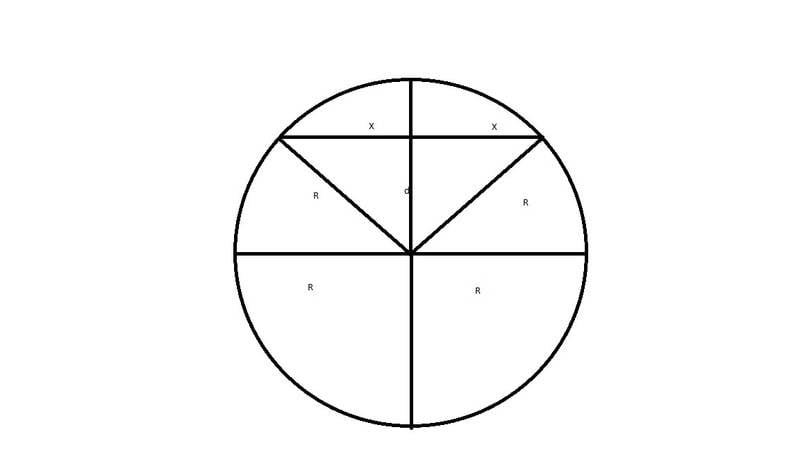# Gauss' Law: Charged Rod & Sphere (Electric Flux)

Const@ntine

## Homework Statement

A charged, straight line/rod of infinite length has a Discrete uniform distribution of charge, has a linear density of λ and is at a distance d from a sphere with a radius of R.Find the entirety of the Electrical Flux that is caused by this charged rod, which passes through the surface of the sphere.

a) When R < d
b) When R > d

## Homework Equations

[/B]
ΦΕ = ∫E⋅dA (for a surface)

ΦΕ = qinternal0 (Gauss' Law)

E = ke∫(dq/r2)r (the r here is a Euclidean Vector)

λ = Q/l

## The Attempt at a Solution

a) Okay, so we know that we have an Electrical Flux only when there is a charge inside the sphere/surrounding shape, because otherwise all the lines of the Electric Field go through one point of the shape, and leave through another, resulting in ΦΕ = 0.

Because R < d, the rod/line is outside the sphere, and thus what we described above happens.

β) Here's where I'm stuck. My initial idea was:

-I'll find E.
-Then I'll use the ΦΕ = ∫E⋅dA (for a surface)

Problem is, I'm not sure how to tackle the finding E part. All the exercises I did on that part where with a straight rod on the x axis. Basically I'm having trouble with doing the integration at E = ke∫dq/r2r

I'm having a bit of trouble with all the integrals and whatnot here, so I could use a nudge or two. Electrics is entirely new to me so I don't have anywhere to run back to, like with previous semesters.

Any help is appreciated!

#### Attachments

Homework Helper
Gold Member
When R > d, can you find the charge enclosed inside the spherical surface?

Homework Helper
Gold Member
You don't need to compute ## E ##. Trying to compute the flux that way and evaluating the surface integral would be extremely difficult. The result can be determined much easier: ## \int E \cdot dA=\frac{Q_{enclosed}}{\epsilon_o} ##. For ## R>d ##, it is a geometry problem to compute the charge enclosed by the sphere, but readily workable.

•Const@ntine
Const@ntine
When R > d, can you find the charge enclosed inside the spherical surface?
You don't need to compute ## E ##. Trying to compute the flux that way and evaluating the surface integral would be extremely difficult. The result can be determined much easier: ## \int E \cdot dA=\frac{Q_{enclosed}}{\epsilon_o} ##. For ## R>d ##, it is a geometry problem to compute the charge enclosed by the sphere, but readily workable.
Sorry for not responding earlier, yesterday was a tough day.

Ah, yeah, now I see it.(I drew a sketch, but the phone's acting up and not sending the pic through email, so I just did a crude drawing in Paint)

From each triangle we have: R2 = d2 + x2 <=> x = √(R2 - d2)

We also know that if we have a Discrete uniform distribution of charge, Q = λ*L.

So Qinternal = λ*(x+x) = 2λ√(R2 - d2)

And thus ΦΕ = 2λ√(R2 - d2)/ε0

That's it, right? That's the book's answer.

#### Attachments

Last edited:
•••